Recently I worked together with intern from Imperial College over the tracking system of COMET for two months, and here I'm going to briefly sum our (impressing) results. To begin with, let's explain what this experiment about.

From physics courses we know that there are conservation laws, most famous are conservation of energy and momenta, but there are other. For example, conservation of different leptonic family numbers.

For instance, electronic number is number of electrons + number of electronic neutrinos. For some time these numbers (electronic number, muonic number, tauonic number) were considered to be preserved, but in the late 1960's it was proved, that neutrino oscillations change the leptonic number of system (neutrino can become electronic from muonic, for instance). This observation is called lepton flavour violation. However, in the Standard Model overall leptonic number (which is sum of three named family numbers) is conserved.

Since that time physicists are searching for other processes which change the leptonic number and include charged leptons (electrons, muons, tauons), which could be a key for new physics, however no success in this direction and the results are currently mostly upper limits we can prove for some processes. Such possible processes have the name of CLFV (charged lepton flavour violation).

### Details of COMET experiment

COMET is small experiment (which is only in plans at this moment) built to detect one specific decay (muon to electron conversion on nucleus), which is suppressed in the standard model:
$$\mu^{-} + N \to e^{-} + N.$$

The frequency of this process is extremely small in SM: it's probability is about $10^{-52}$, which in particular means that we are sure this will not happen during the experiment.

COMET is sending lots of muons (with fixed energy) on aluminum, and makes the muons to interact with it. The process we are looking for is
$$\mu^{-} + Al \to e^{-} + Al,$$
while this isn't the only way we can obtain electron from muon
$$\mu^{-} \to e^{-} + \nu_{\mu} + \overline{\nu}_e.$$

Pay attention,  that second process is 'normal' in the sense it doesn't violate the leptonic conservation laws.

The only way to determine whether we had decay-in-orbit (DIO) or not is to measure the energy of resulting electron (since neutrinos are very elusive particles and we are unable to detect it). Fortunately, the distributions of energies of electron is quite different for these processes and background electrons usually have lower energy.Distribution of energies for signal process and main background (Decay-in-orbit) in COMET

### Some other illustrations of COMET experimentThe scheme of planned experiment COMET. Detector (with aluminum target) will be in the end of this pipeline. This is what planned during phase II, but for the first time experiment will be simpler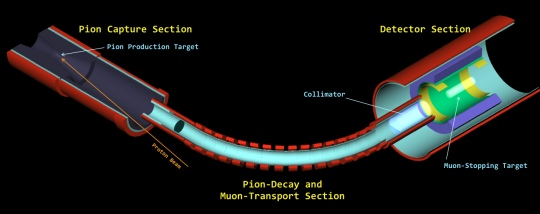COMET during phase I will be much simpler.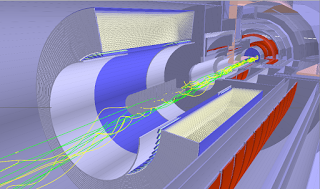Detector of COMET (CyDET). The detector is filled with sensitive wires (white). When muon hits the aluminum target (in the center), the electron produced travels over helix trajectories of larger radius in magnetic field and hits wires. The radius of trajectory depends on the transverse momentum of electron.

### Data collected from COMET

The main information (apart one from trigger) is the data taken from wires, which are registering when charged particle flies nearby (by adsorbing the particles after ionization) and also it measures the time when this happened.

Another characteristic, which helps in distinguishing electrons, is the radius of trajectory, which is computed (in constant magnetic field) by formula
$$r = \dfrac{p_T}{eB},$$
so for electron we know the distribution of gyroradius from distribution of energies. Gyroradius is how we actually will reconstruct energy of electron.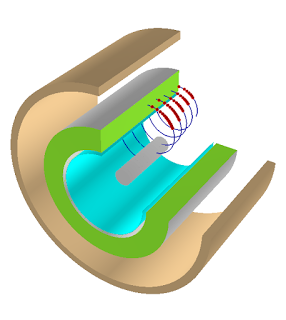Typical signal event in COMET, particle is helixing in magnetic field, leaving energy in drift cambers.

In orthogonal projection of CyDET, the collected information from wires will look like this: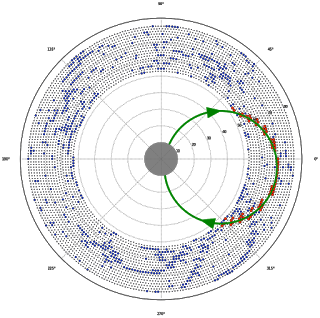Typical picture of signal event in experiment. Red dots are corresponding to signal hits and form a circle of needed radius. Also one can see here many background hits, which come from other particles flying through detector.

But the shape of circle with some radius is not the only useful information. During signal hits usually less energy is disposed (which may sound a bit contradictory to what I wrote earlier, but the faster particle flies, the less energy it disposes).The energy deposited is very strong feature, though leaves significant amount of bck hits, which can spoil the picture, when we have high occupancy.

Apart from this, we can use the 'hit time' (measured from the moment when trigger detected particle).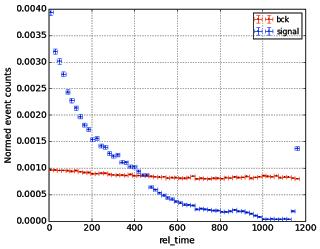The time after trigger tends to be smaller, while background hits are distributed uniformly across the time. This is actually to be checked with better monte-carlo simulations (which are quite poor at the moment).

The main goal of our part is classification of signal vs background hits.

In the next post I'll write about how we applied machine learning to this problem and achieved promising results.

PS. There is second part of this post, devoted to machine learning.